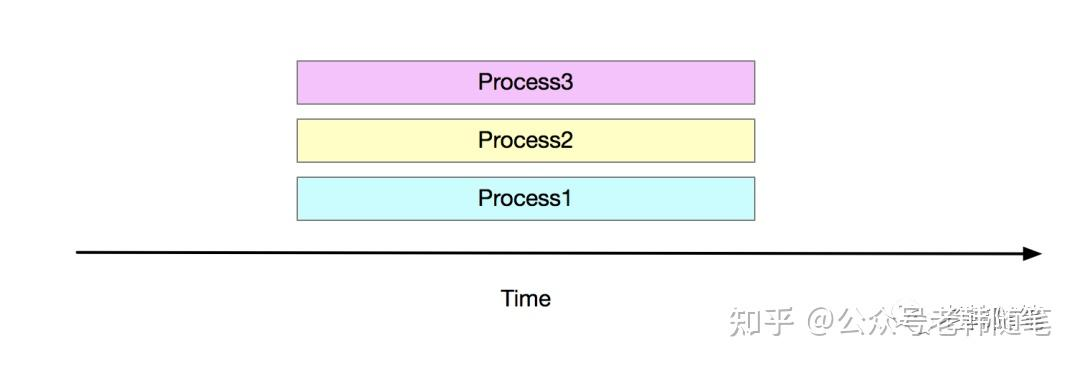# Python并发编程

##python中的多进程(multi-processing)是Python中的并行的实现形式。

```import time

def process(work):
time.sleep(2)
print('process {}'.format(work))

def process_works(works):
for work in works:
process(work)

def main():
works = [
'work1',
'work2',
'work3',
'work4'
]
start_time = time.time()
process_works(works)
end_time = time.time()
print('use {} seconds'.format(end_time - start_time))

if __name__ == '__main__':
main()

##输出##
process work1
process work2
process work3
process work4
use 8.016737222671509 seconds
```

• 先是遍历任务列表；
• 然后对当前任务进行操作；
• 等到当前操作完成后，再对下一个任务进行同样的操作，一直到结束。

```import time
import concurrent.futures

def process(work):
time.sleep(2)
print('process {} '.format(work))

def process_works(works):
executor.map(process, works)

def main():
works = [
'work1',
'work2',
'work3',
'work4'
]
start_time = time.time()
process_works(works)
end_time = time.time()
print('use {} seconds'.format(end_time - start_time))

if __name__ == '__main__':
main()

####输出####
process work1
process work2
process work3
process work4

use 2.006268262863159 seconds
```
`可以看到耗时用了2s多，一下子效率提升了4倍。我们来分析一下下面这段代码。`
```with concurrent.futures.ThreadPoolExecutor(max_workers=4) as executor:
executor.map(process, works)
```

```import asyncio
import time

async def process(work):
await asyncio.sleep(2)
print('process {}'.format(work))

async def process_works(works):

def main():
works = [
'work1',
'work2',
'work3',
'work4'
]
start_time = time.time()
asyncio.run(process_works(works))
end_time = time.time()
print('use {} seconds'.format(end_time - start_time))

if __name__ == '__main__':
main()

####输出####
process work1
process work2
process work3
process work4
use 2.0058629512786865 seconds
```

• 如何I/O负载高，并且I/O操作很慢，需要很多任务/线程协同实现，那么使用 Asyncio 更合适。
• 如何I/O负载高，并且I/O操作很快，只需要有限数量的任务/线程，那么使用多线程就可以了。